# Compressible Analogs of Riemann S-Type Ellipsoids

Here we attempt to develop a self-consistent-field type, iterative technique that will permit the construction of steady-state structures that are compressible analogs of Riemann S-Type (incompressible) ellipsoids. We will build upon the recent work of Ou (2006).

As viewed from a rotating frame of reference and written in Eulerian form, the steady-state version of the three-dimensional principal governing equations are: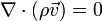$\nabla\cdot(\rho \vec{v}) = 0$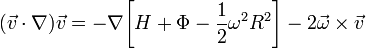$(\vec{v}\cdot \nabla)\vec{v} = -\nabla \biggl[H + \Phi -\frac{1}{2}\omega^2 R^2 \biggr] -2\vec{\omega}\times\vec{v}$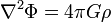$\nabla^2 \Phi = 4\pi G \rho$

## Proposed Solution Strategy

### Preamble:

Specify the three "polar" boundary locations, a,b, and c; specify the direction but not the magnitude of the rotating frame's angular velocity, for example,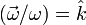$(\vec{\omega}/\omega) = \hat{k}$; pin the central density to the value ρc = 1. Define the following dimensionless density, velocity vector, angular velocity, enthalpy, gravitational potential, and position vector: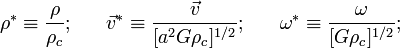$\rho^* \equiv \frac{\rho}{\rho_c} ; ~~~~~{\vec{v}}^* \equiv \frac{\vec{v}}{[a^2G\rho_c]^{1/2}} ; ~~~~~\omega^* \equiv \frac{\omega}{[G\rho_c]^{1/2}} ;$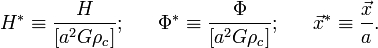$H^* \equiv \frac{H}{[a^2G\rho_c]} ; ~~~~~\Phi^* \equiv \frac{\Phi}{[a^2G\rho_c]} ; ~~~~~{\vec{x}}^* \equiv \frac{\vec{x}}{a} .$

From here, on, spatial operators are assumed to be in terms of the dimensionless coordinates.

### Step #1:

Guess a 3D density distribution — such as a uniform-density ellipsoid, or one of the converged models from Ou (2006) — that conforms to a selected set of positional boundary conditions, that is, where the density goes to zero along the three principal axes at x = a, y = b, and z = c. Solve the Poisson equation in order to derive values for Φ everywhere inside and on the surface of the 3D configuration: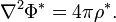$\nabla^2 \Phi^* = 4\pi \rho^* .$

### Step #2:

Use the continuity equation and the curl of the Euler equation to numerically derive the structure but not the overall magnitude of the velocity flow-field throughout the 3D configuration. Take advantage of the fact that the direction,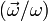$(\vec{\omega}/\omega)$, has been specified; and assume that the 3D density distribution is known. Here are the relevant equations: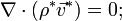$\nabla\cdot(\rho^* {\vec{v}}^*) = 0 ;$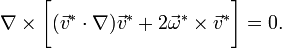$\nabla\times \biggl[({\vec{v}}^*\cdot \nabla){\vec{v}}^* +2 {\vec{\omega}}^* \times {\vec{v}}^* \biggr] = 0 .$

The first of these is a scalar equation; the second is a vector equation and it will presumably provide two useful scalar equations (perhaps constraining the two components of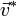${\vec{v}}^*$ that are perpendicular to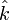$\hat{k}$ ?). Since the left-hand-side of the second equation is obviously nonlinear in the velocity, we may have to linearize this set of equations and look for small "corrections"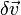$\delta\vec{v}$ to an initial "guess" for the velocity field, such as the flow field in Riemann S-type ellipsoids, which is also the flow-field adopted by Ou (2006).

### Step #3:

Take the divergence of the Euler equation and use it to solve for H throughout the configuration, given the structure of the flow-field obtained in Step #2. Boundary conditions at the three "poles" of the configuration may suffice to uniquely determine ω, the overall normalization factor for the flow-field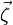$\vec\zeta$ — hopefully this is analogous to solving for the vorticity parameter λ in Ou (2006) — and the Bernoulli constant (or something equivalent). The relevant "Poisson"-like equation is: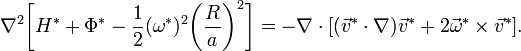$\nabla^2 \biggl[H^* + \Phi^* -\frac{1}{2}(\omega^*)^2 \biggl(\frac{R}{a}\biggr)^2 \biggr] = - \nabla\cdot [({\vec{v}}^*\cdot \nabla){\vec{v}}^* + 2 {\vec{\omega}}^*\times {\vec{v}}^* ] .$

## Example of Riemann S-Type Ellipsoids

### Preamble

First, set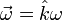${\vec{\omega}} = \hat{k}\omega$ and vz = 0, and write out the Cartesian components of the vector,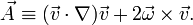$\vec{A} \equiv ({\vec{v}}\cdot \nabla){\vec{v}} +2 {\vec{\omega}} \times {\vec{v}} .$

The components are: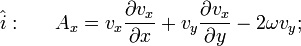$~~~~~\hat{i}:~~~~~A_x = v_x \frac{\partial v_x}{\partial x} + v_y \frac{\partial v_x}{\partial y} -2\omega v_y ;$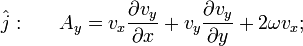$~~~~~\hat{j}:~~~~~A_y = v_x \frac{\partial v_y}{\partial x} + v_y \frac{\partial v_y}{\partial y} +2\omega v_x ;$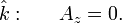$~~~~~\hat{k}:~~~~~A_z = 0 .$

The curl of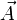$\vec{A}$ (needed in Step #2, above) produces a vector with the following three Cartesian components: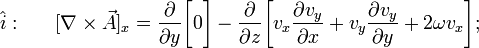$~~~~~\hat{i}:~~~~~[\nabla\times\vec{A}]_x = \frac{\partial}{\partial y} \biggl[0 \biggr] - \frac{\partial}{\partial z} \biggl[ v_x \frac{\partial v_y}{\partial x} + v_y \frac{\partial v_y}{\partial y} +2\omega v_x \biggr] ;$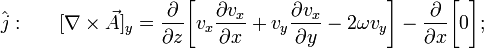$~~~~~\hat{j}:~~~~~[\nabla\times\vec{A}]_y = \frac{\partial}{\partial z} \biggl[ v_x \frac{\partial v_x}{\partial x} + v_y \frac{\partial v_x}{\partial y} -2\omega v_y \biggr] - \frac{\partial}{\partial x} \biggl[0 \biggr] ;$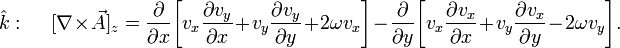$~~~~~\hat{k}:~~~~~[\nabla\times\vec{A}]_z = \frac{\partial}{\partial x} \biggl[ v_x \frac{\partial v_y}{\partial x} + v_y \frac{\partial v_y}{\partial y} +2\omega v_x \biggr] - \frac{\partial}{\partial y} \biggl[ v_x \frac{\partial v_x}{\partial x} + v_y \frac{\partial v_x}{\partial y} -2\omega v_y \biggr] .$

Hence, demanding (as in Step #2) that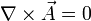$\nabla\times\vec{A} = 0$ means that each of these components independently must be zero. This, in turn, implies: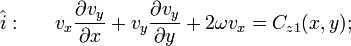$~~~~~\hat{i}:~~~~~ v_x \frac{\partial v_y}{\partial x} + v_y \frac{\partial v_y}{\partial y} +2\omega v_x = C_{z1}(x,y);$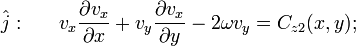$~~~~~\hat{j}:~~~~~ v_x \frac{\partial v_x}{\partial x} + v_y \frac{\partial v_x}{\partial y} -2\omega v_y = C_{z2}(x,y) ;$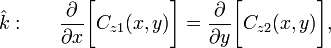$~~~~~\hat{k}:~~~~~ \frac{\partial}{\partial x} \biggl[ C_{z1}(x,y) \biggr] = \frac{\partial}{\partial y} \biggl[C_{z2}(x,y) \biggr] ,$

where the integration "constants" Cz1 and Cz2 may be functions of x and/or y but they must be independent of z.

Generically, the continuity equation demands,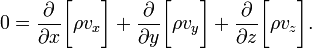$0 = \frac{\partial}{\partial x}\biggl[ \rho v_x \biggr] + \frac{\partial}{\partial y}\biggl[ \rho v_y \biggr] + \frac{\partial}{\partial z}\biggl[ \rho v_z \biggr] .$

The divergence of$\vec{A}$ (providing the right-hand-side of the Poisson-like equation in Step #3, above) generates: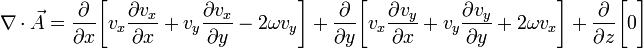$\nabla\cdot\vec{A} = \frac{\partial}{\partial x} \biggl[ v_x \frac{\partial v_x}{\partial x} + v_y \frac{\partial v_x}{\partial y} -2\omega v_y \biggr] + \frac{\partial}{\partial y} \biggl[ v_x \frac{\partial v_y}{\partial x} + v_y \frac{\partial v_y}{\partial y} +2\omega v_x \biggr] + \frac{\partial}{\partial z} \biggl[ 0 \biggr]$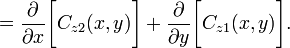$= \frac{\partial}{\partial x} \biggl[ C_{z2}(x,y) \biggr] + \frac{\partial}{\partial y} \biggl[C_{z1}(x,y) \biggr] .$

### Riemann Flow-Field

In Riemann S-Type ellipsoids, the adopted planar flow-field as viewed from the rotating reference frame is,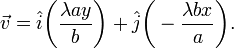$\vec{v} = \hat{i} \biggl( \frac{\lambda a y}{b} \biggr) + \hat{j} \biggl( - \frac{\lambda b x}{a} \biggr) .$

Hence,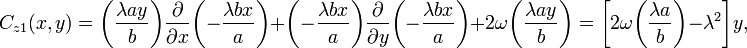$C_{z1}(x,y) = \biggl( \frac{\lambda a y}{b} \biggr) \frac{\partial}{\partial x}\biggl( - \frac{\lambda b x}{a} \biggr) + \biggl( - \frac{\lambda b x}{a} \biggr) \frac{\partial}{\partial y}\biggl( - \frac{\lambda b x}{a} \biggr) +2\omega \biggl( \frac{\lambda a y}{b} \biggr) = \biggl[2\omega\biggl( \frac{\lambda a }{b} \biggr)- \lambda^2 \biggr]y,$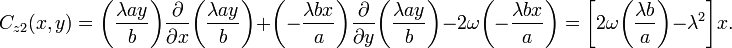$C_{z2}(x,y) = \biggl( \frac{\lambda a y}{b} \biggr) \frac{\partial}{\partial x}\biggl( \frac{\lambda a y}{b} \biggr) + \biggl( - \frac{\lambda b x}{a} \biggr) \frac{\partial}{\partial y}\biggl( \frac{\lambda a y}{b} \biggr) -2\omega \biggl( - \frac{\lambda b x}{a} \biggr) = \biggl[2\omega\biggl( \frac{\lambda b }{a} \biggr) - \lambda^2 \biggr]x .$

Because Cz1 is independent of x and Cz2 is independent of y, we see that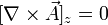$[\nabla\times\vec{A}]_z = 0$, trivially. With this specified velocity flow-field and the appreciation that Riemann S-type ellipsoids also have uniform density, the continuity equation is also trivially satisfied; specifically,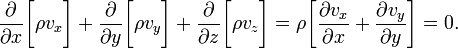$\frac{\partial}{\partial x}\biggl[ \rho v_x \biggr] + \frac{\partial}{\partial y}\biggl[ \rho v_y \biggr] + \frac{\partial}{\partial z}\biggl[ \rho v_z \biggr] = \rho \biggl[ \frac{\partial v_x}{\partial x} + \frac{\partial v_y}{\partial y} \biggr] = 0 .$

However, the right-hand-side of our Poisson-like equation is not zero; rather, it is,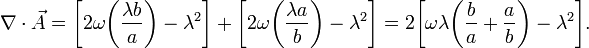$\nabla\cdot\vec{A} = \biggl[2\omega\biggl( \frac{\lambda b }{a} \biggr) - \lambda^2 \biggr] + \biggl[2\omega\biggl( \frac{\lambda a }{b} \biggr)- \lambda^2 \biggr] = 2 \biggl[\omega\lambda \biggl( \frac{b}{a} + \frac{a}{b} \biggr) - \lambda^2 \biggr].$

### Summary

What can we learn from the Riemann S-Type ellipsoids? Well, let's assume that the structure of our equilibrium flow-field will be more complicated than simply "flow along elliptical paths", but let's continue to assume that a solution can be found in which the flow remains planar, that is, vz = 0 everywhere. Also, let's continue to align the rotation axis of the frame with the z-axis of the configuration. The three steps in our proposed solution strategy become:

#### Step #1 (simplified):

Guess a 3D density distribution where the density goes to zero along the three principal axes at x = a, y = b, and z = c. Solve the Poisson equation in order to derive values for Φ everywhere inside and on the surface of the 3D configuration: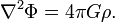$\nabla^2 \Phi = 4\pi G \rho .$

#### Step #2 (simplified):

Use the continuity equation and the curl of the Euler equation to numerically derive the structure but not the overall magnitude of the velocity flow-field throughout the 3D configuration, assuming$\vec{\omega}=\hat{k}\omega$ and the 3D density distribution is known. Here are the relevant equations: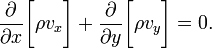$\frac{\partial}{\partial x}\biggl[ \rho v_x \biggr] + \frac{\partial}{\partial y}\biggl[ \rho v_y \biggr] = 0 .$$~~~~~\hat{i}:~~~~~ v_x \frac{\partial v_y}{\partial x} + v_y \frac{\partial v_y}{\partial y} +2\omega v_x = C_{z1}(x,y);$$~~~~~\hat{j}:~~~~~ v_x \frac{\partial v_x}{\partial x} + v_y \frac{\partial v_x}{\partial y} -2\omega v_y = C_{z2}(x,y) ;$$~~~~~\hat{k}:~~~~~ \frac{\partial}{\partial x} \biggl[ C_{z1}(x,y) \biggr] = \frac{\partial}{\partial y} \biggl[C_{z2}(x,y) \biggr] ,$

Now, for the adopted flow-field of the Riemann ellipsoids, Cz1(x,y) is only a function of y and Cz2(x,y) is only a function of x — that is,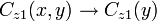$C_{z1}(x,y) \rightarrow C_{z1}(y)$ and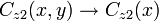$C_{z2}(x,y) \rightarrow C_{z2}(x)$. Hence, the third ($\hat{k}$) condition is automatically satisfied. I don't know whether or not we will find that our more general velocity fields exhibit the same nice character.

#### Step #3 (simplified):

Take the divergence of the Euler equation and use it to solve for H throughout the configuration, given the structure of the flow-field obtained in Step #2. The relevant "Poisson"-like equation is: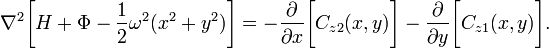$\nabla^2 \biggl[H + \Phi -\frac{1}{2}\omega^2 (x^2 + y^2) \biggr] = - \frac{\partial}{\partial x} \biggl[ C_{z2}(x,y) \biggr] - \frac{\partial}{\partial y} \biggl[C_{z1}(x,y) \biggr] .$

In the Riemann ellipsoids, the RHS of this expression is a constant. More importantly, in the Riemann case, it is possible to bring the constants from Step #2 inside the spatial operator on the LHS and establish the following algebraic condition: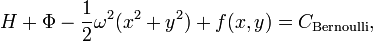$H + \Phi -\frac{1}{2}\omega^2 (x^2 + y^2) + f(x,y) = C_\mathrm{Bernoulli} ,$

where the function f(x,y) contains only quadratic terms in x and y. Specifically [see Eq. (6) of Ou (2006)],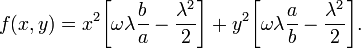$f(x,y) = x^2 \biggl[\omega\lambda \frac{b}{a}-\frac{\lambda^2}{2} \biggr] + y^2 \biggl[ \omega\lambda \frac{a}{b}-\frac{\lambda^2}{2} \biggr] .$

This makes sense because in the Riemann case the equipotential surfaces were perfect ellipsoids, just like the shape of the centrifugal potential term. So the remaining "source" terms could adopt the same "shape". But in our more general case, surfaces of constant Φ will have more complicated shapes, so it will be necessary for the function f(x,y) (or equivalent) to incorporate higher order terms in x and y. Is it possible that equilibrium configurations can be found by including additional terms just in x4 and y4?

## A Related Two-dimensional Treatment

### Preamble

In a related work, Korycansky & Papaloizou (1996, ApJS, 105, 181; hereafter KP96) developed a method to find nontrivial, nonaxisymmetric steady-state flows in a two-dimensional setting. Specifically, they constructed infinitesimally thin steady-state disk structures in the presence of a time-independent, nonaxisymmetric perturbing potential. While their problem was only two-dimensional and they did not seek a self-consistent solution of the gravitational Poisson equation, the approach they took to solving the 2D Euler equation in tandem with the continuity equation for a compressible fluid may very well be instructive. What follows is a summary of their approach.

As in our above preamble to discussion of Riemann S-Type Ellipsoids, KP96 set${\vec{\omega}} = \hat{k}\omega$. Hence, their steady-state Euler equation and steady-state continuity equation become (see their Eq. 1 or their Eq. 7),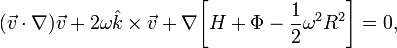$(\vec{v}\cdot \nabla)\vec{v} + 2\omega\hat{k}\times\vec{v} + \nabla \biggl[H + \Phi -\frac{1}{2}\omega^2 R^2 \biggr] = 0 ,$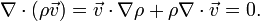$\nabla\cdot(\rho \vec{v}) = \vec{v}\cdot\nabla\rho + \rho\nabla\cdot\vec{v} = 0 .$

Note that the KP96 notation is slightly different from ours:

• Σ is used in place of ρ to denote a two-dimensional surface density;
• Ω is used instead of ω to denote the angular frequency of the rotating reference frame;
• W is used instead of H to denote the fluid enthalpy; and
• Φg represents the combined, time-independent gravitational and centrifugal potential, that is, Φg = (Φ − ω2R2 / 2).

Using the vector identity,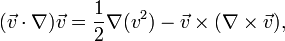$(\vec{v}\cdot \nabla)\vec{v} = \frac{1}{2}\nabla(v^2) - \vec{v}\times(\nabla\times\vec{v}) ,$

the above steady-state Euler equation can also be written as,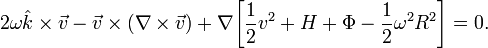$2\omega\hat{k}\times\vec{v} - \vec{v}\times(\nabla\times\vec{v}) + \nabla \biggl[\frac{1}{2}v^2 + H + \Phi -\frac{1}{2}\omega^2 R^2 \biggr] = 0 .$

Up to this point, no assumptions have been made regarding the behavior of the vector flow-field; we have only chosen to align the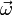$\vec{\omega}$ with the coordinate unit vector,$\hat{k}$. In particular, these derived forms for the steady-state Euler and continuity equations can serve to describe a fully 3D problem.

Before proceeding further we should emphasize that, in the context of the Euler equation written in this form (i.e., the form preferred by KP96), the vector$\vec{A}$ defined in the preamble, above, should be written,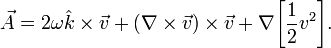$\vec{A} = 2\omega\hat{k}\times\vec{v} +(\nabla\times\vec{v})\times\vec{v} + \nabla \biggl[\frac{1}{2}v^2 \biggr] .$

### No Vertical Motions

Now we restrict the flow by setting vz = 0, that is, from here on we will assume that all the motion is planar. Also, following the lead of KP96, we define the vorticity of the fluid,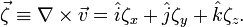$\vec{\zeta} \equiv \nabla\times\vec{v} = \hat{i}\zeta_x + \hat{j}\zeta_y + \hat{k}\zeta_z .$

[Note that (unfortunately) KP96 use ω instead of ζ to represent the rotating-frame vorticity.] In terms of the components of the vorticity vector, the steady-state Euler equation therefore becomes,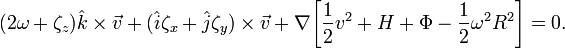$(2\omega + \zeta_z)\hat{k}\times\vec{v} + (\hat{i}\zeta_x + \hat{j}\zeta_y)\times\vec{v} + \nabla \biggl[\frac{1}{2}v^2 + H + \Phi -\frac{1}{2}\omega^2 R^2 \biggr] = 0 .$

Continuing to follow the lead KP96, we next take the curl of this Euler equation. Because the curl of a gradient is always zero, this leads us to the same condition discussed above — but this time written in terms of the components of the vorticity — namely,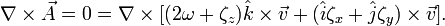$\nabla\times\vec{A} = 0 = \nabla\times [(2\omega + \zeta_z)\hat{k}\times\vec{v} + (\hat{i}\zeta_x + \hat{j}\zeta_y)\times\vec{v}] .$

Using another vector identity, namely,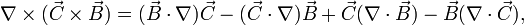$\nabla\times(\vec{C} \times \vec{B}) = (\vec{B}\cdot\nabla)\vec{C} - (\vec{C}\cdot\nabla)\vec{B} + \vec{C}(\nabla\cdot\vec{B}) - \vec{B}(\nabla\cdot\vec{C}),$

and remembering that we are assuming vz = 0, we see in this case that the vector condition$\nabla\times\vec{A}=0$ leads to the following three independent scalar constraints: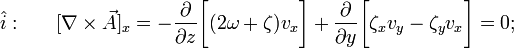$~~~~~\hat{i}:~~~~~ [\nabla\times\vec{A}]_x = - \frac{\partial }{\partial z}\biggl[ (2\omega + \zeta)v_x \biggr] + \frac{\partial}{\partial y} \biggl[ \zeta_x v_y - \zeta_y v_x \biggr] = 0 ;$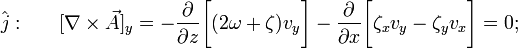$~~~~~\hat{j}:~~~~~ [\nabla\times\vec{A}]_y = - \frac{\partial }{\partial z} \biggl[ (2\omega + \zeta)v_y \biggr] - \frac{\partial}{\partial x} \biggl[ \zeta_x v_y - \zeta_y v_x \biggr] = 0 ;$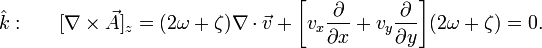$~~~~~\hat{k}:~~~~~ [\nabla\times\vec{A}]_z = (2\omega + \zeta)\nabla\cdot\vec{v} + \biggl[ v_x \frac{\partial}{\partial x} + v_y \frac{\partial}{\partial y} \biggr](2\omega + \zeta) = 0 .$

With the understanding that, by definition,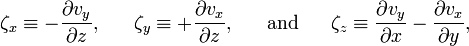$\zeta_x \equiv - \frac{\partial v_y}{\partial z} , ~~~~~ \zeta_y \equiv + \frac{\partial v_x}{\partial z} , ~~~~~ \mathrm{and} ~~~~~ \zeta_z \equiv \frac{\partial v_y}{\partial x} - \frac{\partial v_x}{\partial y} ,$

it can be shown that these three constraints are identical to the ones presented in the preamble, above.

### Solution Strategy

Constraint #1: For their two-dimensional disk problem, KP96 focused on the constraint provided by the z-component of the curl of the Euler equation, which can be rewritten as (see above derivation, or Eq. 2 of KP96),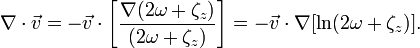$\nabla\cdot\vec{v} =-\vec{v} \cdot \biggl[ \frac{\nabla(2\omega + \zeta_z)}{(2\omega + \zeta_z)} \biggr] = -\vec{v} \cdot \nabla[\ln(2\omega + \zeta_z)].$

Constraint #2: But from the continuity equation they also know that,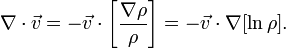$\nabla\cdot\vec{v} = -\vec{v}\cdot\biggl[\frac{\nabla\rho}{\rho} \biggr] = -\vec{v} \cdot \nabla[\ln\rho] .$

Hence,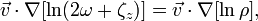$\vec{v} \cdot \nabla[\ln(2\omega + \zeta_z)] = \vec{v} \cdot \nabla[\ln\rho] ,$

that is,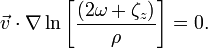$\vec{v} \cdot \nabla\ln\biggl[ \frac{(2\omega + \zeta_z)}{\rho} \biggr] = 0 .$

This is essentially KP96's Eq. (3).

Introduce stream function: The constraint implied by the continuity equation also suggests that it might be useful to define a stream function in terms of the momentum density — instead of in terms of just the velocity, which is the natural treatment in the context of incompressible fluid flows. KP96 do this. They define the stream function, Ψ, such that (see their Eq. 4),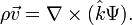$\rho\vec{v} = \nabla\times(\hat{k}\Psi) .$

in which case,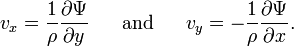$v_x = \frac{1}{\rho} \frac{\partial \Psi}{\partial y} ~~~~~\mathrm{and}~~~~~ v_y = - \frac{1}{\rho} \frac{\partial \Psi}{\partial x} .$

This implies as well that the z-component of the fluid vorticity can be expressed in terms of the stream function as follows (see Eq. 5 of KP96):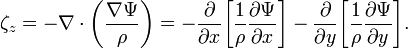$\zeta_z = - \nabla\cdot \biggl( \frac{\nabla\Psi}{\rho} \biggr) = - \frac{\partial}{\partial x} \biggl[ \frac{1}{\rho} \frac{\partial\Psi}{\partial x} \biggr] - \frac{\partial}{\partial y} \biggl[ \frac{1}{\rho} \frac{\partial\Psi}{\partial y} \biggr].$

According to KP96, this expression, taken in combination with the conclusion drawn above from the second constraint — that is, Eq. (3) taken in combination with Eq. (4) from KP96 — "tell us that the 'vortensity' z + 2ω) / ρ is constant along streamlines which are lines of constant Ψ." The vortensity is therefore a function of Ψ alone, so we can write,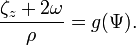$\frac{\zeta_z + 2\omega}{\rho} = g(\Psi) .$

Constraint #3:

Taking the scalar product of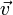$\vec{v}$ and the following form of the steady-state Euler equation,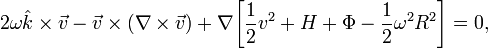$2\omega\hat{k}\times\vec{v} - \vec{v}\times(\nabla\times\vec{v}) + \nabla \biggl[\frac{1}{2}v^2 + H + \Phi -\frac{1}{2}\omega^2 R^2 \biggr] = 0 ,$

we obtain the constraint,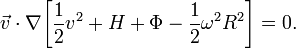$\vec{v}\cdot\nabla \biggl[\frac{1}{2}v^2 + H + \Phi -\frac{1}{2}\omega^2 R^2 \biggr] = 0 .$

When tied with our earlier discussion, this means that the Bernoulli function also must be constant along streamlines. Hence, we can write,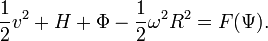$\frac{1}{2}v^2 + H + \Phi -\frac{1}{2}\omega^2 R^2 = F(\Psi) .$

KP96 then go on to demonstrate that the relationship between the functions g(Ψ) and F(Ψ) is,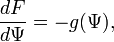$\frac{dF}{d\Psi} = -g(\Psi) ,$

which allows the determination of F up to a constant of integration.

### Summary

In summary, KP96 constrain their flow as follows:

1. They use the z-component of the curl of the Euler equation;
2. They use the compressible version of the continuity equation;
3. Instead of taking the divergence of the Euler equation to obtain a Poisson-like equation, they obtain an algebraic constraint on the Bernoulli function (as in our traditional SCF technique) by simply "dotting"$\vec{v}$ into the Euler equation.

 © 2014 - 2020 by Joel E. Tohline |   H_Book Home   |   YouTube   | Appendices: | Equations | Variables | References | Ramblings | Images | myphys.lsu | ADS |